## LetsPlayMaths.Com

WELCOME TO THE WORLD OF MATHEMATICS

Class 2 Recap

## Class 2 Recap

In Standard two, we learnt how to add 3-digits numbers with or without carrying. Few examples are shown below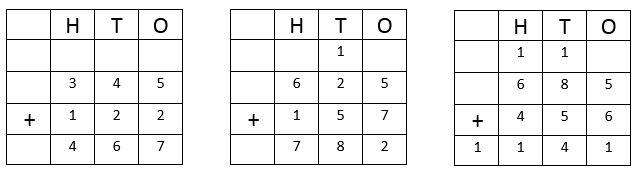The same 3-digit numbers addition method is applied to 4-digits, 5-digits and 6-digits. Let us learn 4-digits
or 5-digits addition without carrying. Few examples are given below.

Example 1. Add 5345 and 4122

Solution. Putting the numbers in tabular format as shown below.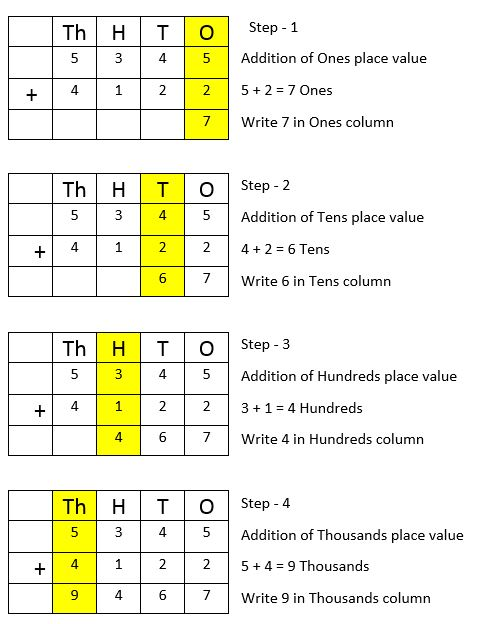So, Sum of 5345 and 4122 is 9467

Example 2. Write in tabular format and add: 34635, 50231 and 3100.

Solution. Putting the numbers in the column form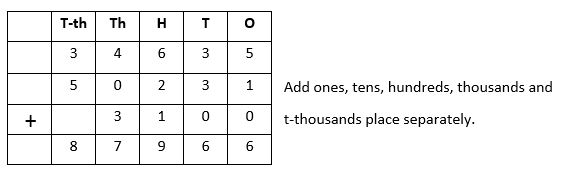We have already learnt the addition on 2 and 3 digit numbers. We will use the same method for addition 4 and 5 digit numbers.

Example 1. Find the sum of 4745 and 4387.

Solution. Putting the numbers in column format as shown below.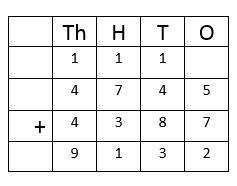Step 1: Add the ones place value numbers. 5 + 7 = 12 Ones = 1 Ten + 2 ones.

Write 2 under ones column and carry 1 ten to the tens place column.

Step 2: Add the tens. 8 + 4 + 1 (Carryover) = 13 Tens = 1 hundred + 3 tens

Write 3 under tens column and carry 1 hundred to the hundreds column.

Step 3: Add the hundreds. 7 + 3 + 1 (Carryover) = 11 hundreds = 1 Thousand + 1 hundred

Write 1 under hundreds column and carry 1 thousands to thousands column.

Step 4: Add the Thousands. 4 + 4 + 1 (Carryover) = 9 thousands

Example 2. Find the sum of 15625, 26472 and 37928

Solution. Putting the numbers in column format as shown below.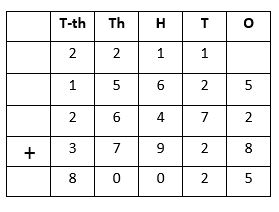In our day to day life we use concept of addition in many ways, few examples are shown below.

Example 1. A salesman sold \$ 4567 on Monday and on Tuesday he sold \$ 3928. Find out how much he sold on these days?

Solution.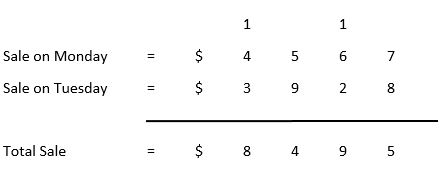So, the total sale is \$ 8495.

Example 1.Population of Philadelphia state is 89765 and population of Ohio state is 67569. What is the total population of both the sates?

Solution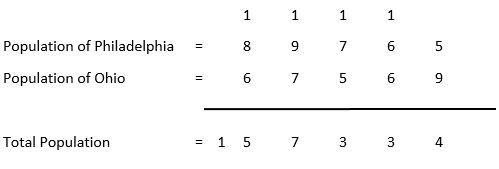So, the total population of both the cities are 157334.